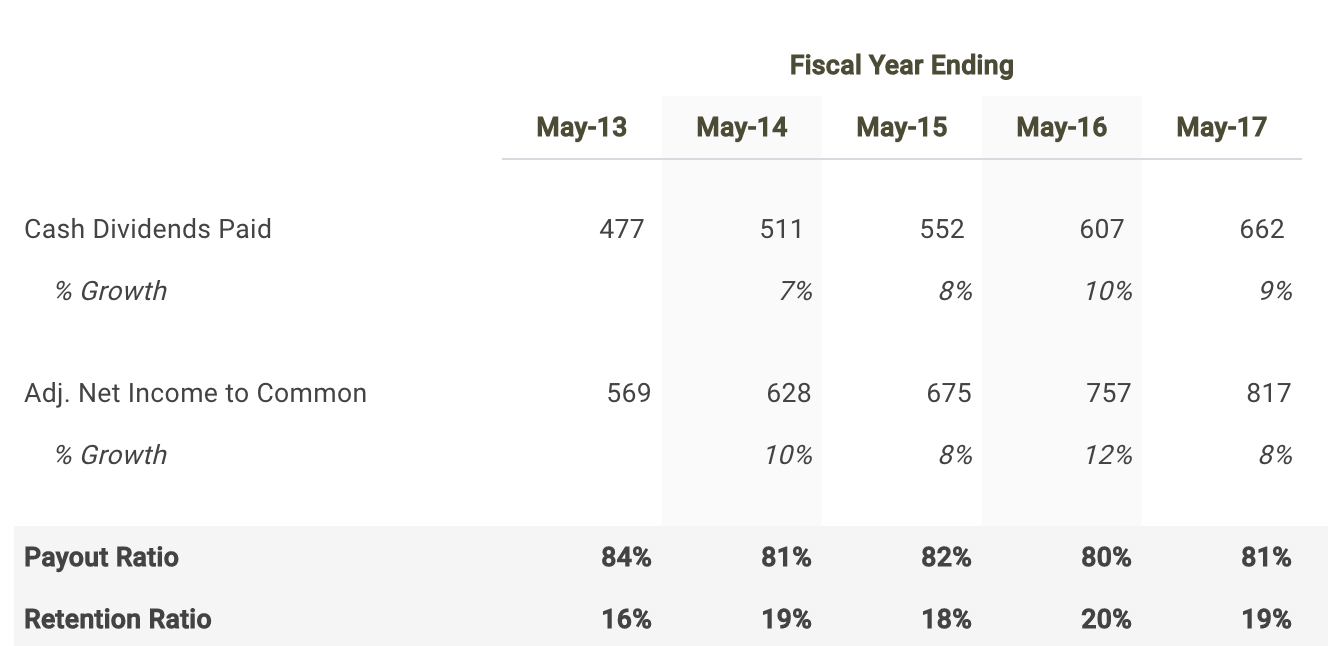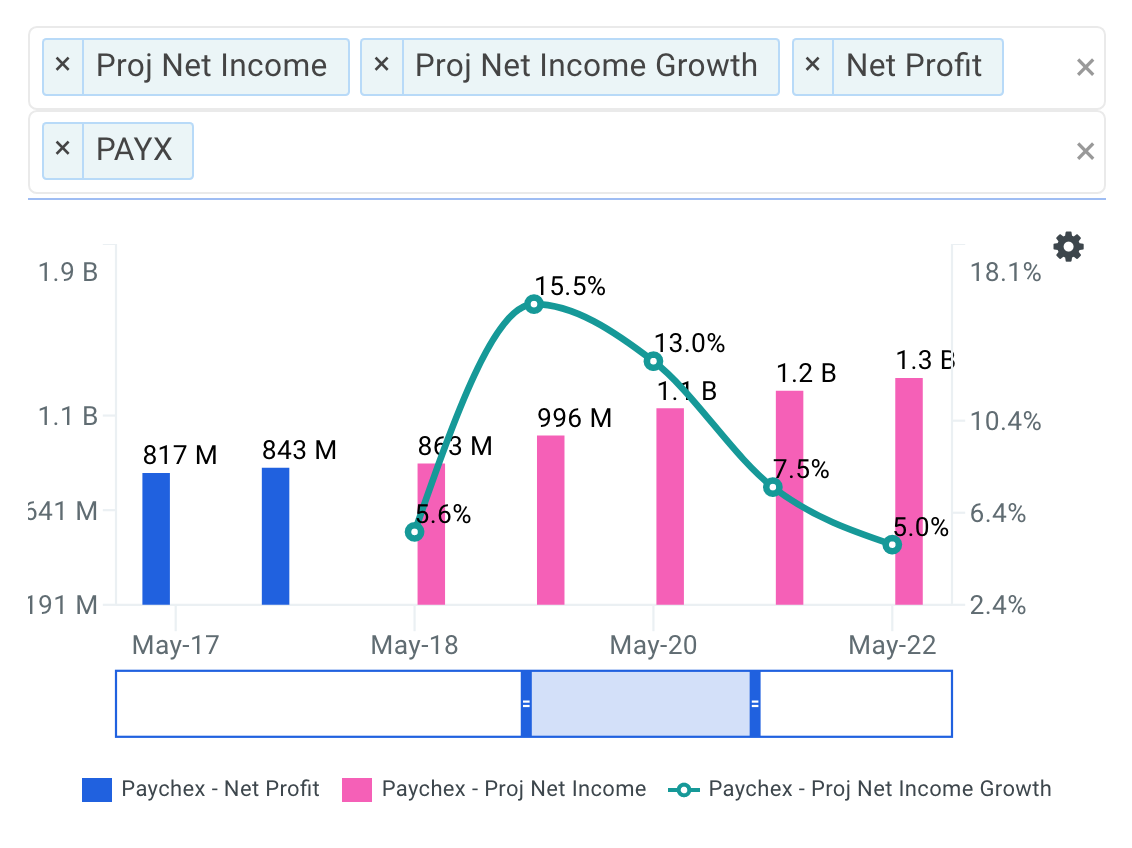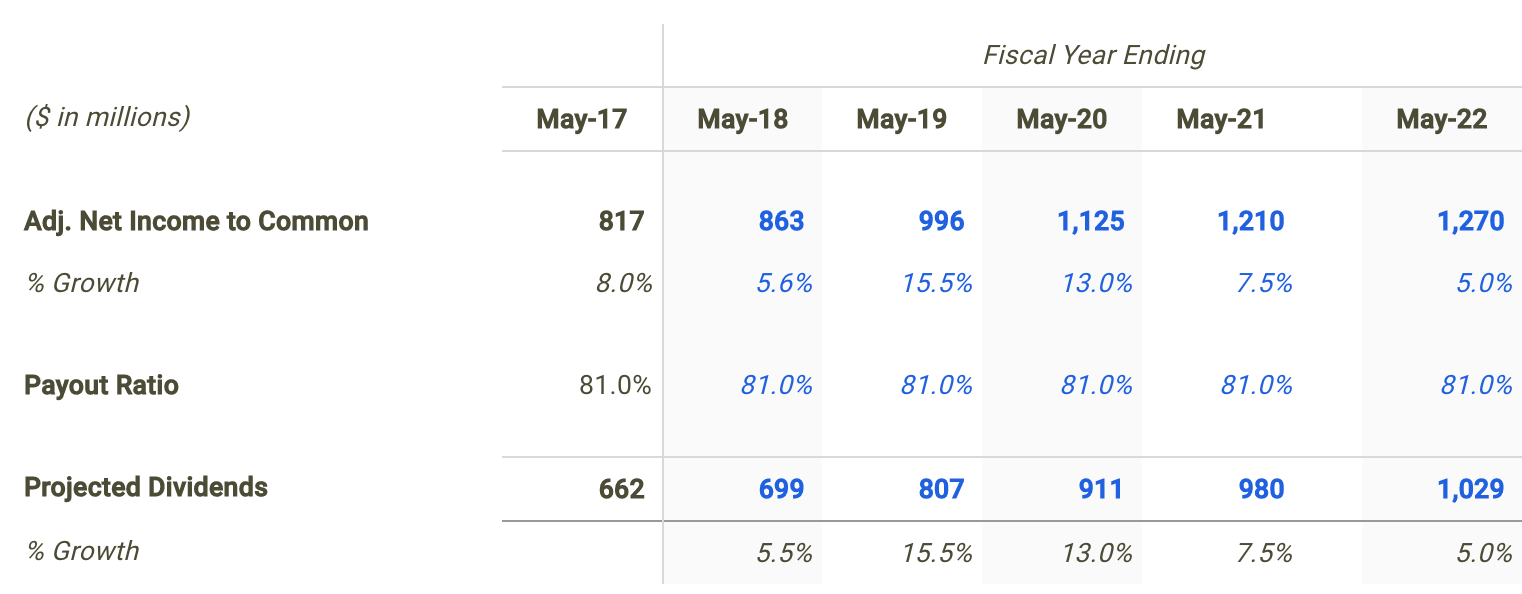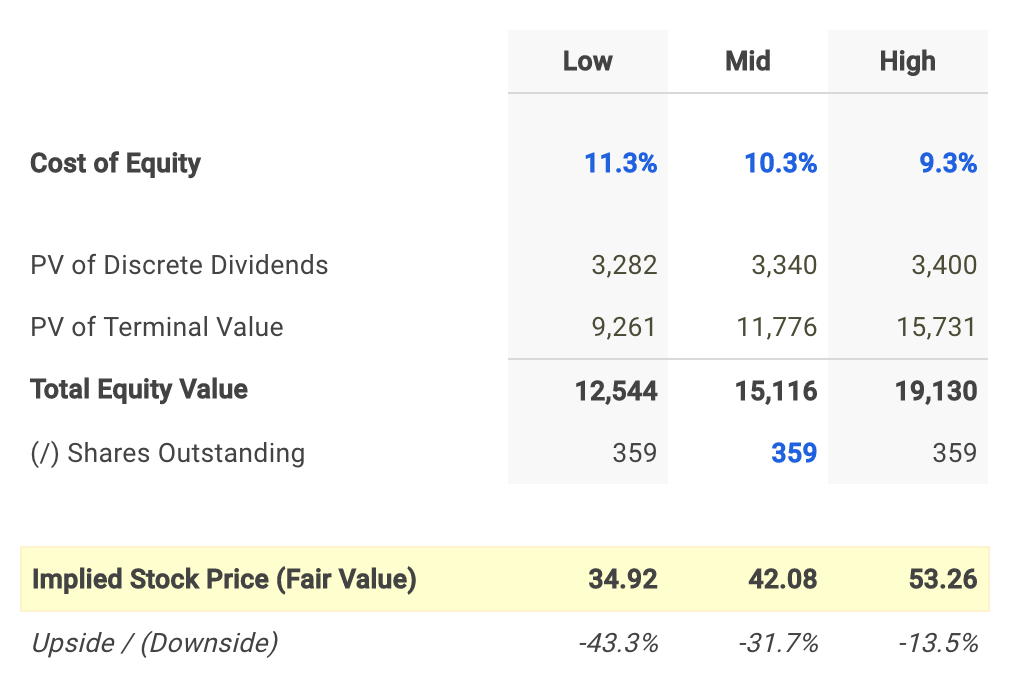Paychex, Inc. (NASDAQ: PAYX) currently pays a dividend yield of 3.2% which is above the Information Technology sector median of 1.7%. While this makes the total return potential for Paychex attractive, investors may change their mind when analyzing the company's future dividends. In this article, I calculate Paychex's fair value by forecasting its dividend distributions and discounting them back to today's value.

##### Valuation Methodologies Are Not Made Equally

The Dividend Discount Model (DDM) estimates the value of a company's stock price based on the theory that its worth is equal to the sum of the present value of its future dividend payments to shareholders.

But before discussing the assumptions used in my dividend discount model for Paychex, it's first helpful to determine if this is actually an appropriate technique to be used when estimating its fair value. Many analysts find it difficult when trying to figure out the correct valuation methodology for a given company or are biased towards one specific approach. This is often a mistake which can negatively impact investment decisions and result in trading losses or missed opportunities. No two companies are the same and every business consists of unique characteristics that may require you to adjust your analysis.

Understanding leverage trends is the first step when determining what valuation analyses are relevant for a given company. When a company's leverage doesn’t fluctuate or is expected to remain stable over time, then an equity valuation model (e.g. equity DCF, DDM) will be the most appropriate valuation technique. The reason for this is because when leverage is stable, interest expense on debt can typically be projected with much more reliability.

How do we check if a company's leverage has been fluctuating or is expected to do so? This isn't always straightforward but checking debt ratio trends over the last three to five years can be a good indicator. Paychex has historically carried no debt on its balance sheet suggesting an equity valuation model is a suitable technique. Now does it make sense to use a dividend discount model knowing that an equity valuation technique is an appropriate methodology?

The next step is to figure out if Paychex pays a dividend and if so, is its payout ratio relatively high (typically above 80%)? The table below highlights this information.source: PAYX dividend discount model

Paychex distributed a total of \$662 million in cash dividends to shareholders in its most recent fiscal year and represented a payout ratio of 81%. It appears that the company meets both criteria. Therefore, it is fitting to use a dividend discount model when determining the fair value of Paychex stock.

##### Forecasting Paychex's Dividends

The first step in building a dividend discount model is to forecast net income since forecasting dividends directly can be difficult. So let's create a net income forecast for the next five years and use the forecast as the basis for future dividends.

As of March 30th, Wall Street analysts are projecting a healthy growth rate in the company's bottom-line over the next five years. Net Income is expected to reach \$1.3 billion by fiscal year 2022.source: PAYX Projected Net Income Growth

I use the net income projections above to serve as the basis for my dividend forecast. The next step is to forecast the payout ratio which I simply kept at its most recent 81% for the next five years.

My dividend forecast for Paychex is shown in the table below:source: PAYX dividend discount model

##### Calculating Paychex's Fair Value

The last step is to select a discount rate to calculate the present value of the forecasted dividends. I used finbox.io’s Weighted Average Cost of Capital (WACC) model to help arrive at an estimate for the company's cost of equity.

I determined a reasonable discount rate for Paychex to be 10.25% at the midpoint. An updated cost of capital analysis using real-time data can be found at finbox.io's Paychex WACC Model Page.The assumptions used in the dividend discount model calculate a fair value per share for Paychex of \$42.08, 31.7% below it's current stock price of \$61.59. Therefore, PAYX appears to be an overvalued stock and not neccesarily a good entry point opportunity.

##### Conclusion: Dividends Don't Support Stock Price

Discovering the fair value of a company can sometimes be difficult. However, determining an appropriate valuation methodology should not be. Knowing when and when not to use the dividend discount model will help in your investment decision making process.

However, it's important to understand that a dividend discount model will inherently undervalue a stock. This is typically the result of the payout ratio assumption being less than 100% implying some cash leakage. Meaning the approach does not capture value that would otherwise build up as cash on the balance sheet. In practice, this excess retained cash is usually paid out to shareholders as special dividends or to make up for cash shortfalls for future dividends during economic downturns.

This helps explain, at least partially, why Paychex looks like an overvalued stock based on this technique. Nevertheless, a dividend discount analysis is still a helpful tool in combination with the understanding that it calculates a conservative intrinsic value estimate. Meaning an extremely attractive opportunity would be a stock where (1) a dividend discount model is appropriate and (2) shares looks undervalued based on its future dividends.

Note that there are a number of fundamental factors I have not considered in this analysis. I recommend that you continue your research on Paychex to gain a better understanding of its future prospects.

##### Author: Matt Hogan

Expertise: Valuation, financial statement analysis

Matt Hogan is also a co-founder of finbox.io. His expertise is in investment decision making. Prior to finbox.io, Matt worked for an investment banking group providing fairness opinions in connection to stock acquisitions. He spent much of his time building valuation models to help clients determine an asset’s fair value. He believes that these same valuation models should be used by all investors before buying or selling a stock.

His work is frequently published at InvestorPlace, Benzinga, ValueWalk, AAII, Barron's, Seeking Alpha and investing.com.

Matt can be reached at matt@finbox.io.

This is not a buy or sell recommendation on any security mentioned.
image source: ncoa